# Observables¶

### Module: RDF¶

class pytim.observables.rdf.RDF(universe, nbins=75, max_radius='full', start=None, stop=None, step=None, observable=None, observable2=None, kargs1=None, kargs2=None)

Calculates a radial distribution function of some observable from two groups.

The two functions must return an array (of scalars or of vectors) having the same size of the group. The scalar product between the two functions is used to weight the distriution function.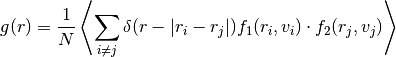Parameters: max_radius (double) – compute the rdf up to this distance. If ‘full’ is supplied (default) computes it up to half of the smallest box side. nbins (int) – number of bins observable (Observable) – observable for the first group observable2 (Observable) – observable for the second group

Example:

>>> import MDAnalysis as mda
>>> import numpy as np
>>> import pytim
>>> from pytim import observables
>>> from pytim.datafiles import *
>>>
>>> u = mda.Universe(WATER_GRO,WATER_XTC)
>>> oxygens = u.select_atoms("name OW")
>>>
>>> nres = observables.NumberOfResidues()
>>>
>>> rdf = observables.RDF(u,nbins=120,        observable=nres,observable2=nres)
>>>
>>> interface = pytim.ITIM(u,alpha=2.,group=oxygens,        cluster_cut=3.5,molecular=False)
>>>
>>> for ts in u.trajectory[::50]:
...     layer=interface.layers[0,0]
...     rdf.sample(layer,layer)
>>> rdf.count=0
>>> np.savetxt('RDF3D.dat', np.column_stack((rdf.bins,rdf.rdf)))


Note that one needs to specify neither both groups, not both observables. If only the first group (observable) is specified, the second is assumed to be the same as the first, as in the following example:

>>> rdf1 = observables.RDF(u,observable=nres)
>>> rdf2 = observables.RDF(u,observable=nres)
>>> rdf3 = observables.RDF(u,observable=nres,observable2=nres)
>>>
>>> rdf1.sample(layer)
>>> rdf2.sample(layer,layer)
>>> rdf3.sample(layer,layer)
>>> print (np.all(rdf1.rdf[:]==rdf2.rdf[:]))
True
>>> print (np.all(rdf1.rdf[:]==rdf3.rdf[:]))
True


## Profiles¶

### Module: Profile¶

class pytim.observables.profile.Profile(direction=None, observable=None, interface=None, symmetry='default', MCnorm=True)

Calculates the profile (normal, or intrinsic) of a given observable across the simulation box.

Parameters: observable (Observable) – ‘Number’, ‘Mass’, or any other observable: calculate the profile of this quantity. If None is supplied, it defaults to the number density. The number density is always calculated on a per atom basis. interface (ITIM) – if provided, calculate the intrinsic profile with respect to the first layers direction (str) – ‘x’,’y’, or ‘z’ : calculate the profile along this direction. (default: ‘z’ or the normal direction of the interface, if provided. MCnorm (bool) – if True (default) use a simple Monte Carlo estimate the effective volumes of the bins.

Example (non-intrinsic, total profile + first 4 layers ):

>>> import numpy as np
>>> import MDAnalysis as mda
>>> import pytim
>>> from   pytim.datafiles import *
>>> from   pytim.observables import Profile
>>>
>>> u = mda.Universe(WATER_GRO,WATER_XTC)
>>> g = u.select_atoms('name OW')
>>> # here we calculate the profiles of oxygens only (note molecular=False)
>>> inter = pytim.ITIM(u,group=g,max_layers=4,centered=True, molecular=False)
>>>
>>> # We create a list of 5 profiles, one for the total and 4 for the first
>>> # 4 layers.
>>> # Note that by default Profile() uses the number of atoms as an observable
>>> Layers = []
>>> for n in range(5):
...     Layers.append(Profile())
>>>
>>> # Go through the trajectory, center the liquid slab and sample the profiles
>>> for ts in u.trajectory[::50]:
...         # this shifts the system so that the center of mass of the liquid slab
...         # is in the middle of the box
...         inter.center()
...
...         Layers.sample(g)
...         Layers.sample(u.atoms[u.atoms.layers == 1 ])
...         Layers.sample(u.atoms[u.atoms.layers == 2 ])
...         Layers.sample(u.atoms[u.atoms.layers == 3 ])
...         Layers.sample(u.atoms[u.atoms.layers == 4 ])
>>>
>>> density=[]
>>> for L in Layers:
...     low,up,avg = L.get_values(binwidth=0.5)
...     density.append(avg)
>>>
>>> # (low + up )/2 is the middle of the bin
>>> np.savetxt('profile.dat',list(zip(low,up,density,density,density,density,density)))


This results in the following profile (sampling more often and zooming close to the interface border)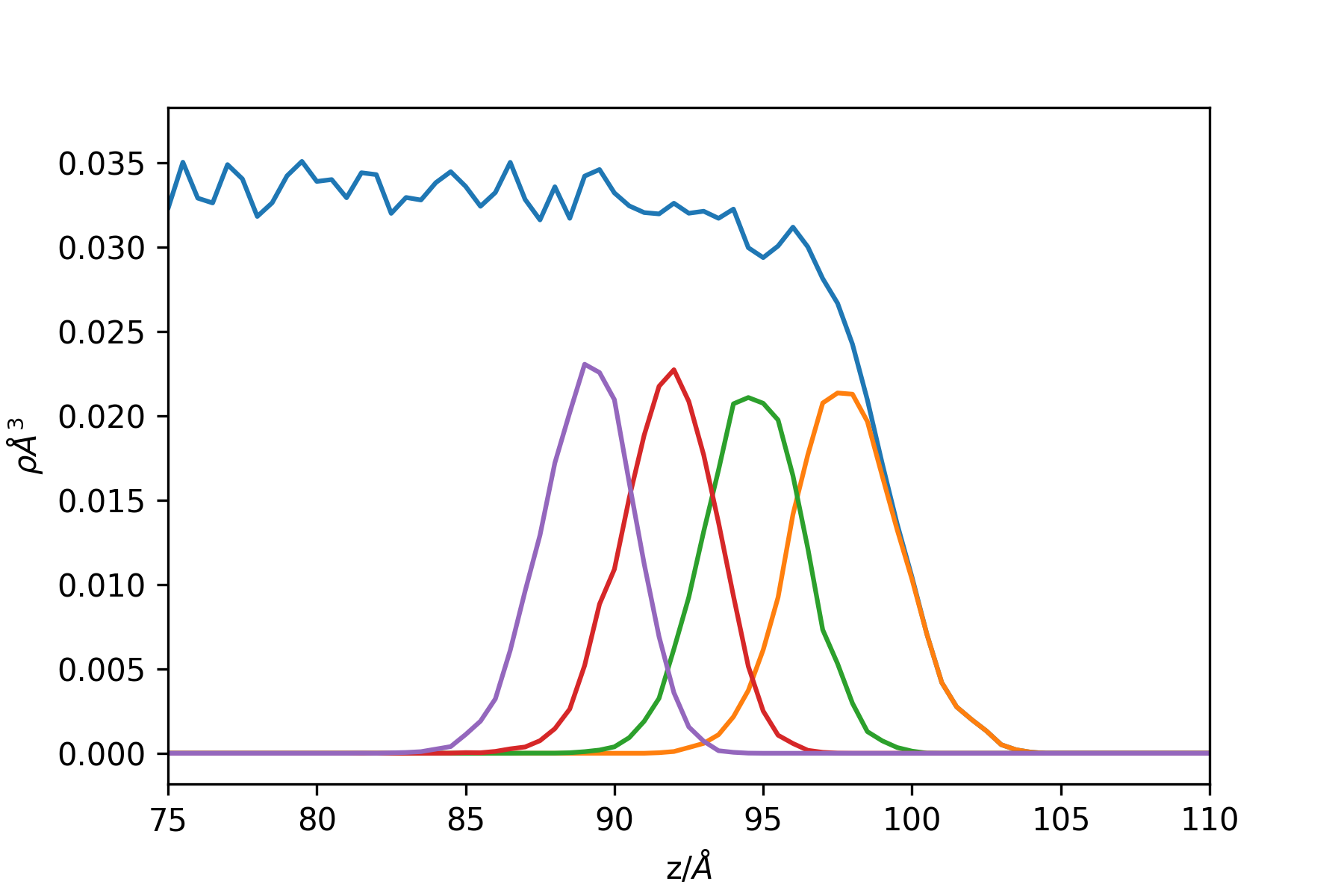Example: the intrinsic profile of a LJ liquid/vapour interface:

>>> import numpy as np
>>> import MDAnalysis as mda
>>> import pytim
>>> from   pytim.datafiles import LJ_GRO
>>> from   pytim.observables import Profile

>>> XTC = pytim.datafiles.pytim_data.fetch('LJ_BIG_XTC',tmpdir='./')
checking presence of a cached copy... not found. Fetching remote file... done.

>>> u = mda.Universe(LJ_GRO,XTC)
>>> g = u.select_atoms('all')
>>>
>>> inter = pytim.ITIM(u,alpha=2.5,cluster_cut=4.5)
>>> profile = Profile(interface=inter)
>>>
>>> for ts in u.trajectory[::50]:
...     profile.sample(g)
>>>
>>> low, up, avg = profile.get_values(binwidth=0.5)
>>> np.savetxt('profile.dat',list(zip(low,up,avg)))


This results in the following profile (sampling more often):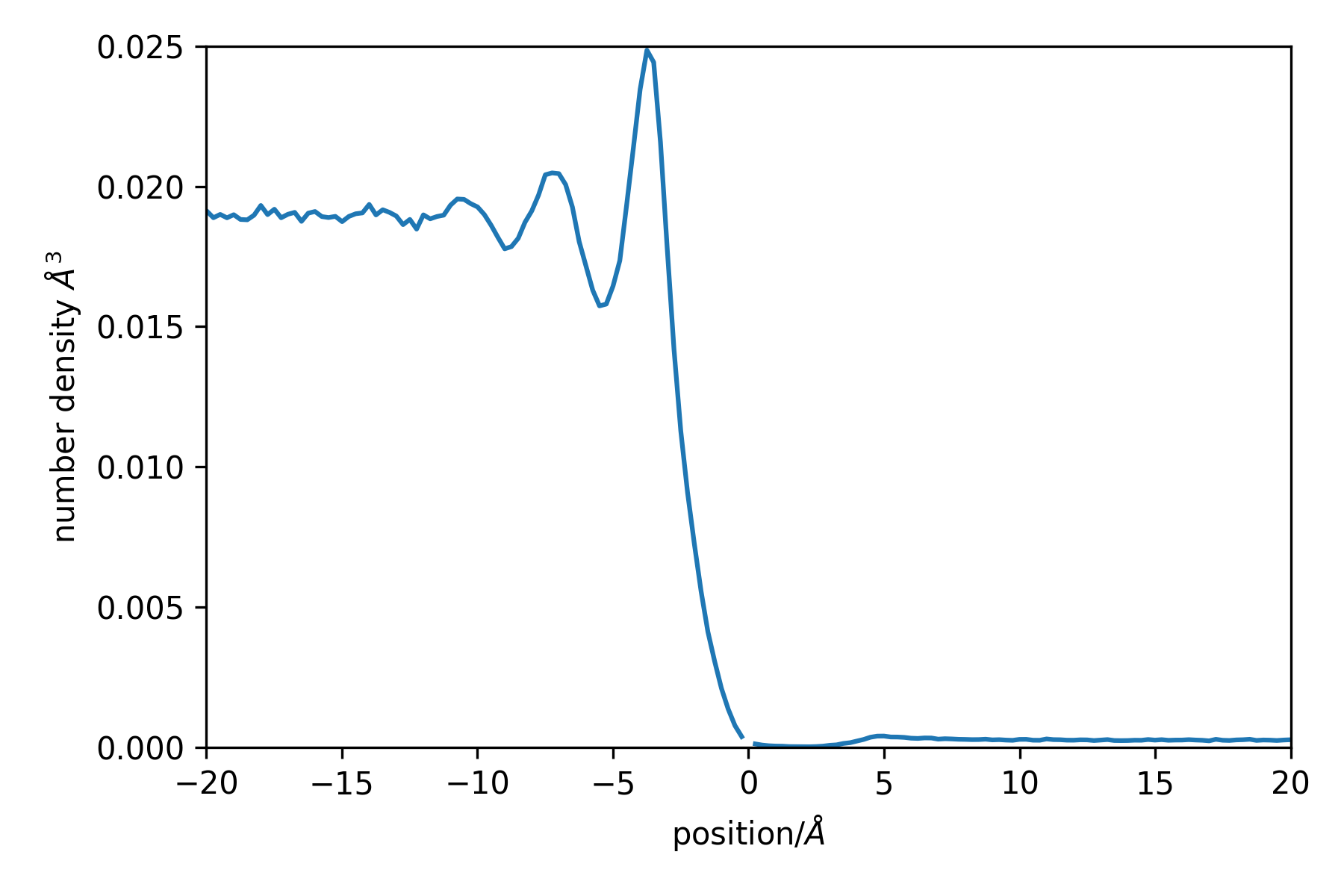Note the missing point at position = 0, this is the delta-function contirbution. Negative positions are within the liquid phase, while positive ones are in the vapour phase.

## Time Correlation Functions¶

class pytim.observables.Correlator(universe=None, observable=None, reference=None)

Computes the (self) correlation of an observable (scalar or vector)

Parameters: observable (Observable) – compute the autocorrelation of this observable. If the observable is None and the reference is not, the survival probability is computed. normalize (bool) – normalize the correlation to 1 at t=0 reference (AtomGroup) – if the group passed to the sample() function changes its composition along the trajectory (such as a layer group), a reference group that includes all atoms that could appear in the variable group must be passed, in order to provide a proper normalization. See the example below.

Example:

>>> import pytim
>>> import MDAnalysis as mda
>>> import numpy as np
>>> from pytim.datafiles import WATERSMALL_GRO
>>> from pytim.utilities import lap
>>> #  tmpdir here is specified only for travis
>>> WATERSMALL_TRR = pytim.datafiles.pytim_data.fetch('WATERSMALL_LONG_TRR',tmpdir='./')
checking presence of a cached copy...

>>> u = mda.Universe(WATERSMALL_GRO,WATERSMALL_TRR)
>>> g = u.select_atoms('name OW')

>>> velocity = pytim.observables.Velocity()
>>> corr = pytim.observables.Correlator(observable=velocity)
>>> for t in u.trajectory[1:]:
...     corr.sample(g)
>>> vacf = corr.correlation()


This produces the following (steps of 1 fs):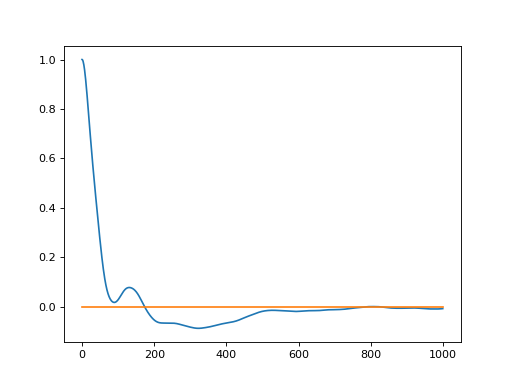In order to compute the correlation for variable groups, one should proceed as follows:

>>> corr = pytim.observables.Correlator(observable=velocity,reference=g)
>>> # notice the molecular=False switch, in order for the
>>> # layer group to be made of oxygen atoms only and match
>>> # the reference group
>>> inter = pytim.ITIM(u,group=g,alpha=2.0,molecular=False)
>>> # example only: sample longer for smooth results
>>> for t in u.trajectory[1:10]:
...     corr.sample(inter.atoms)
>>> layer_vacf = corr.correlation()


In order to compute the survival probability of some atoms in a layer, it is possible to pass observable=None together with the reference group:

>>> corr = pytim.observables.Correlator(observable=None, reference = g)
>>> inter = pytim.ITIM(u,group=g,alpha=2.0, molecular=False)
>>>  # example only: sample longer for smooth results
>>> for t in u.trajectory[1:10]:
...     corr.sample(inter.atoms)
>>> survival = corr.correlation()

correlation(normalized=True, continuous=True)

Calculate the autocorrelation from the sampled data

Parameters: normalized (bool) – normalize the correlation function to: its zero-time value for regular correlations; to the average of the characteristic function for the survival probability. continuous (bool) – applies only when a reference group has been specified: if True (default) the contribution of a particle at time lag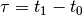is considered only if the particle did not leave the reference group between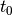and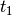. If False, the intermittent correlation is calculated, and the above restriction is released.

Example:

>>> # We build a fake trajectory to test the various options:
>>> import MDAnalysis as mda
>>> import pytim
>>> import numpy as np
>>> from pytim.datafiles import WATER_GRO
>>> from pytim.observables import Correlator, Velocity
>>> np.set_printoptions(suppress=True,precision=3)
>>>
>>> u = mda.Universe(WATER_GRO)
>>> g = u.atoms[0:2]
>>> g.velocities*=0.0
>>> g.velocities+=1.0
>>>
>>> # velocity autocorrelation along x, variable group
>>> vv = Correlator(observable=Velocity('x'), reference=g)
>>> nn = Correlator(reference=g) # survival probability in group g
>>>
>>> for c in [vv,nn]:
...     c.sample(g)     # t=0
...     c.sample(g)     # t=1
...     c.sample(g[:1]) # t=2, exclude the second particle
...     g.velocities /= 2. # from now on v=0.5
...     c.sample(g)     # t=3
>>>


The timeseries sampled can be accessed using:

>>> print(vv.timeseries) # rows refer to time, columns to particle
[[1.0, 1.0], [1.0, 1.0], [1.0, 0.0], [0.5, 0.5]]
>>>
>>> print(nn.timeseries)
[[True, True], [True, True], [True, False], [True, True]]
>>>


Note that the average of the characteristic function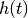is done over all trajectories, including those that start with h=0. The correlation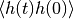is divided by the average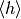computed over all trajectores that extend up to a time lag. The normalize switch has no effect.

>>> # normalized, continuous
>>> corr = nn.correlation()
>>> print (np.allclose(corr, [ 7./7, 4./5, 2./4, 1./2]))
True
>>> # normalized, intermittent
>>> corr = nn.correlation(continuous=False)
>>> print (np.allclose(corr, [ 7./7, 4./5, 3./4, 2./2 ]))
True


The autocorrelation functions are calculated by taking into account in the average only those trajectory that start with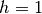(i.e., which start within the reference group). The normalization is done by dividing the correlation at time lagby its value at time lag 0 computed over all trajectories that extend up to time lagand do not start with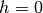.

>>> # not normalizd, intermittent
>>> corr = vv.correlation(normalized=False,continuous=False)
>>> c0 = (1+1+1+0.25+1+1+0.25)/7
>>> c1 = (1+1+0.5+1)/5 ; c2 = (1+0.5+0.5)/4 ; c3 = (0.5+0.5)/2
>>> print (np.allclose(corr, [ c0, c1, c2, c3]))
True
>>> # check normalization
>>> np.all(vv.correlation(continuous=False) == corr/corr)
True
>>> # not normalizd, continuous
>>> corr = vv.correlation(normalized=False,continuous=True)
>>> c0 = (1+1+1+0.25+1+1+0.25)/7
>>> c1 = (1+1+0.5+1)/5 ; c2 = (1+0.5)/4 ; c3 = (0.5+0.)/2
>>> print (np.allclose(corr, [ c0, c1, c2, c3]))
True
>>> # check normalization
>>> np.all(vv.correlation(continuous=True) == corr/corr)
True

sample(group)

Sample the timeseries for the autocorrelation function

Parameters: group (AtomGroup) – compute the observable using this group

## Misc¶

class pytim.observables.LayerTriangulation(interface, return_triangulation=True, return_statistics=True)

Computes the triangulation of the surface and some associated quantities. Notice that this forces the interface to be centered in the box.

Parameters: universe (Universe) – the MDAnalysis universe interface (ITIM) – compute the triangulation with respect to it layer (int) – (default: 1) compute the triangulation with respect to this layer of the interface return_triangulation (bool) – (default: True) return the Delaunay triangulation used for the interpolation return_statistics (bool) – (default: True) return the Delaunay triangulation used for the interpolation Observable LayerTriangulation

Example:

>>> import pytim
>>> import MDAnalysis as mda
>>> from pytim.datafiles import WATER_GRO

>>> interface = pytim.ITIM(mda.Universe(WATER_GRO),molecular=False)
>>> surface   = pytim.observables.LayerTriangulation(                            interface,return_triangulation=False)
>>> stats     = surface.compute()
>>> print ("Surface= {:04.0f} A^2".format(stats))
Surface= 6328 A^2

class pytim.observables.IntrinsicDistance(interface, symmetry='default')

Initialize the intrinsic distance calculation.

Parameters: interface (PYTIM) – compute the intrinsic distance with respect to this interface symmetry (str) – force calculation using this symmetry, if availabe (e.g. ‘generic’, ‘planar’, ‘spherical’) If ‘default’, uses the symmetry selected in the PYTIM interface instance.

Example:

>>> import MDAnalysis as mda
>>> import pytim
>>> from pytim import observables
>>> from pytim.datafiles import MICELLE_PDB
>>> u = mda.Universe(MICELLE_PDB)
>>> micelle = u.select_atoms('resname DPC')
>>> waterox = u.select_atoms('type O and resname SOL')
>>> inter = pytim.GITIM(u,group=micelle, molecular=False, alpha=2.0)
>>> dist  = observables.IntrinsicDistance(interface=inter)
>>> d = dist.compute(waterox)
>>> print(d)
[25.733  8.579  8.852 ... 18.566 13.709  9.876]

compute(inp, kargs=None)

Compute the intrinsic distance of a set of points from the first layers.

Parameters: positions (ndarray) – compute the intrinsic distance for this set of points

## Basic Observables¶

class pytim.observables.Number(*arg, **kwarg)

The number of atoms.

compute(inp, kargs=None)

Compute the observable.

Parameters: inp (AtomGroup) – the input atom group one, for each atom in the group
class pytim.observables.NumberOfResidues(*arg, **karg)

The number of residues.

Instead of associating 1 to the center of mass of the residue, we associate 1/(number of atoms in residue) to each atom. In an homogeneous system, these two definitions are (on average) equivalent. If the system is not homogeneous, this is not true anymore.

compute(inp, kargs=None)

Compute the observable.

Parameters: inp (AtomGroup) – the input atom group one, for each residue in the group
class pytim.observables.Position(*arg, **kwarg)

Atomic positions

compute(inp, kargs=None)

Compute the observable.

Parameters: inp (AtomGroup) – the input atom group atomic positions
class pytim.observables.Velocity(*arg, **kwarg)

Atomic velocities

compute(inp, kargs=None)

Compute the observable.

Parameters: inp (AtomGroup) – the input atom group atomic velocities
class pytim.observables.Force(*arg, **kwarg)

Atomic forces

compute(inp, kargs=None)

Compute the observable.

Parameters: inp (AtomGroup) – the input atom group atomic forces
class pytim.observables.Orientation(universe, options='')

Orientation of a group of points.

Parameters: options (str) – optional string. If normal is passed, the orientation of the normal vectors is computed If the option ‘molecular’ is passed at initialization the coordinates of the second and third atoms are folded around those of the first.
compute(inp, kargs=None)

Compute the observable.

Parameters: inp (ndarray) – the input atom group. The length be a multiple of three the orientation vectors

For each triplet of positions A1,A2,A3, computes the unit vector between A2-A1 and A3-A1 or, if the option ‘normal’ is passed at initialization, the unit vector normal to the plane spanned by the three vectors.